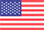CloseMain Categories
Search

# Circuits, Third Edition Textbook

Circuits, Third Edition Textbook helps students understand a wide range of timeless principles and problem techniques that are essential to circuit studies. Generally, circuit theory is the entry course in pursuing education in electrical and computer engineering. While this book is a great introductory text, a background in physics and elementary differential and integral calculus is assumed.

MFR Part #: 784236-01
\$154.00
Availability: In stock

Authors:

Fawwaz Ulaby, University of Michigan
Michel Maharbiz, University of California, Berkeley
Cynthia Furse, University of Utah

ISBN # 978-1-934891-22-3

## Circuits, Third Edition Textbook Highlights

• ?Ten new Technology Briefs (for a total of 32)
• Explains how concepts in each chapter are applied in real-world applications and expose students to the far-reaching nature of the electrical engineering discipline
• Technology Briefs cover applications in circuits, medicine, the physical world, optics, signals and systems, and more

• ?RLC circuits (previous Chapter 6) is now split into two separate chapters:
• One using time domain (Chapter 6)
• The other using the Laplace Transform (Chapter 12)

NI my-DAQ integration

NI my-DAQ is a convenient, portable measurement tool that turns a PC into a basic electrical engineering lab with a DVM, analog and digital power supplies, function generator, oscilloscope, Bode plot analyzer, and diode analyzer.

• 53 integrative end-of-chapter problems
• In addition to solving a circuit problem analytically and simulating its behavior with Multi-sim, a complementary third approach is to physically build the circuit on a circuit board and use the NI my-DAQ to interface the circuit to a computer.
• Student tutorial (Appendix F) for getting started with NI my-DAQ.

• New problems, examples and enhancements throughout the presentation
• The following resources are found on a link provided inside the front cover of the book:
• (1) All Figures and Tables, and many of the major equations
• (2) Solutions to all of the Exercises contained in the book
• (3) 43 Multi-sim Modules (see Appendix C for details)
• (4) Latest NI Multi-sim and LabVIEW Student Edition software via access code download
• (5) MathScriptRT software, which can perform matrix inversion and many other calculations, much like The MathWorks, Inc MATLAB ® software. The basic commands used by MATLAB® also work in MathScript RT Module
• Product can be reviewed only after purchasing it
• Only registered users can write reviews
*
*
• Excellent

Authors:

Fawwaz Ulaby, University of Michigan
Michel Maharbiz, University of California, Berkeley
Cynthia Furse, University of Utah

ISBN # 978-1-934891-22-3

## Circuits, Third Edition Textbook Highlights

• ?Ten new Technology Briefs (for a total of 32)
• Explains how concepts in each chapter are applied in real-world applications and expose students to the far-reaching nature of the electrical engineering discipline
• Technology Briefs cover applications in circuits, medicine, the physical world, optics, signals and systems, and more

• ?RLC circuits (previous Chapter 6) is now split into two separate chapters:
• One using time domain (Chapter 6)
• The other using the Laplace Transform (Chapter 12)

NI my-DAQ integration

NI my-DAQ is a convenient, portable measurement tool that turns a PC into a basic electrical engineering lab with a DVM, analog and digital power supplies, function generator, oscilloscope, Bode plot analyzer, and diode analyzer.

• 53 integrative end-of-chapter problems
• In addition to solving a circuit problem analytically and simulating its behavior with Multi-sim, a complementary third approach is to physically build the circuit on a circuit board and use the NI my-DAQ to interface the circuit to a computer.
• Student tutorial (Appendix F) for getting started with NI my-DAQ.